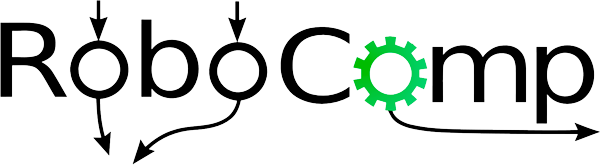11 Jun 2016

# Working with Caffe CPP API:

This tutorial explains the Caffe classification example. Implementations are present in /examples/cpp_classification.

Required inputs:

1. Model file: These is the file describing the layer configuration and its hyperparameters.
2. trained_file: These is the file containing learned weights.
3. mean_file: It is good idea to subtract the mean of all images in dataset. It essentially does background subtraction.
4. label_file: CNN gives prediction probability for all the categories on which it was trained. The label file gives mapping between category id and class name.

Pipeline:

1. set device(CPU/GPU)
2. Load the network net_.reset(new Net(model_file, TEST)); net_->CopyTrainedLayersFrom(trained_file);
3. Load the binaryproto mean file. SetMean(mean_file);
4. Set input and output layer (Note: only single input and output layer supported.) Blob* input_layer = net_->input_blobs(); Blob* output_layer = net_->output_blobs();
5. Compute prediction for given test image. std::vector predictions = classifier.Classify(image); The prediction structure has two components. Prediction.first gives label and Prediction.second gives corresponding score.

The prediction method pipeline is given as follows:

1. Change the dimension of input layer

input_layer->Reshape(1, num_channels_, input_geometry_.height, input_geometry_.width);

2. Forward dimension change to all layers.

``````net_->Reshape();

``````
1. Wrap the input layer of the network in separate cv::Mat objects (one per channel). This way we save one memcpy operation and ww don’t need to rely on cudaMemcpy2D. The last preprocessing operation will write the separate channels directly to the input layer.
``````WrapInputLayer(&input_channels);

``````
1. Convert the input image to the input image format of the network and write the separate BGR planes directly to the input layer of the network.

Preprocess(img, &input_channels);

2. Run forward pass for the network.

net_->ForwardPrefilled();

3. Copy the output layer to a std::vector

Blob* output_layer = net_->output_blobs(); const float* begin = output_layer->cpu_data(); const float* end = begin + output_layer->channels(); return std::vector(begin, end);

Harit Pandya